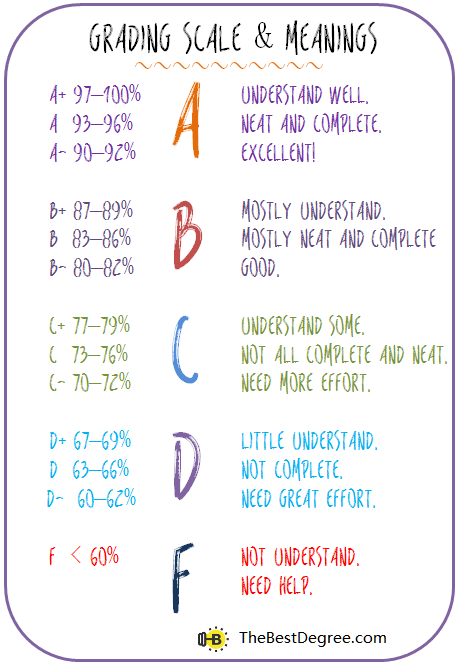0.00

with combined weight of 0.00

To get an overall grade of ( B ), you will need an average grade of 0 on the remaining weight of 0 percent.

## How to calculate weighted gradeA weighted grade or score is average of a set of grades, where each grade (g) carries a different weight (w) of importance. A weighted grade is usually calculated by the following formula:

Weighted grade = (g1×w1+ g2×w2+ g3×w3+...)/(w1+w2+w3...)

For example:

On a syllabus, the percentage of each assignments and exam is given as follow: Homework: 10%, Quizzes: 20%, Essays: 20%, Midterm: 25%, Final: 25%.

The grades earned in each assignment or exam are: Homework: 93%, Quizzes: 85%, Essays: 86%, Midterm: 87%, Final: 91%.

Then using a calculator the overall grade is calculated as:

Overall grade = (93×10%+ 85×20%+ 86×20%+87×25%+ 91×25%)/100% = 88 percent.

In the scenario of before final exam, the average grade is:

Weighted grade = (93×10%+ 85×20%+ 86×20%+87×25%)/(10%+ 20%+ 20%+ 25%) = 87 percent.

To get an overall grade of 88 percent, the final grade need to be:

(88×100%- 87×75%)/25% = 91 percent.